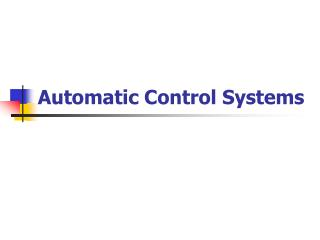DownloadDownload PresentationAutomatic Control Systems

# Automatic Control Systems

Download Presentation## Automatic Control Systems

- - - - - - - - - - - - - - - - - - - - - - - - - - - E N D - - - - - - - - - - - - - - - - - - - - - - - - - - -
##### Presentation Transcript

1. Automatic Control Systems

2. P43 2-6 (a)(e) Find the inverse Laplace transforms of the following functions. Perform partial-fraction expansion on G(s) first ,then use the Laplace transform table. (a) (e)

3. P70 3-8(b) Find the transfer functions Y2/Y1 and Y1/Y2 of the SFGs shown in Fig.3P-8 Fig.3P-8

4. P311 7-13 For the control systems shown in Fig.7P-7, find the values of K and Ktso that the maximum overshoot of the output is approximately 4.3 percent and the rise time tr is approximately 0.2 sec. Simulate the systems with any time-response simulation program to check the accuracy of your solutions. Fig.7P-7

5. P229 6-9 The block diagram of a motor-control system with tachometer feedback is shown in Fig.6P-9.Find the range of the tachometer constant Kt so that the system is asymptotically stable. Fig.6P-9

6. P230 6-12(a) The conventional Routh-Hurwitz criterion gives information only on the location of the zeros of a polynomial F(s) with respect to the left half and right half of the s-place. Devise a linear transformation s=f (p,α) , where p is a complex variable, so that the Routh- Hurwitz criterion can be applied to determine whether F(s) has zeros to the right of the line s=-α,where αis a positive real number. Apply the transformation to the following characteristic equations to determine how many roots are to the right of the line s=-1 in the s-plane. (a)

7. P309 7-3(c) Determine the step, ramp, and parabolic error constants of the following unity-feedback control systems. The forward-path transfer functions are given. (c)

8. P309 7-5 (a) (d) The following transfer functions are given for a single-loop nonunity-feedback control system. Find the steady-state errors due to a unit-step input, a unit-ramp input, and a parabolic input, (a) (d)

9. P347 8-2(a) For the loop transfer functions that follows, find the angle of departure or arrival of the root loci at the designated pole or zero. (a) Angle of arrival (K<0) and angle of departure (K>0)at s=j.

10. P347 8-5(h) Construct root-locus diagram for each of the following control systems for which the poles and zeros of G(s)H(s) are given. The characteristic equation is obtained by equating the numerator of 1+ G(s)H(s) to zero. (h) Poles at 0, 0, -8, -8; no finite zeros.

11. P424 9-9(a) (b) The loop transfer functions L(s) of single –feedback-loop systems are given below. Sketch the Nyquist plot of L(jω) for ω=0 to ω=∞. Determine the stability of the closed-loop system. If the system is unstable, find the number of poles of the closed-loop transfer function that are in the right-half s-plane. Solve for the intersect of L(jω)on the negative real axis of the L(jω)-plane analytically. You may construct the Nyquist plot of L(jω)using any computer program. (a) (b)

12. P428 9-26(c) The forward-path transfer functions of unity-feedback control systems are given in the following. Plot the Bode diagram of G(jω)/K and do the following: (1) Find the value of K so that the gain margin of the system is 20 dB. (2) Find the value of K so that the phase margin of the system is 45o.